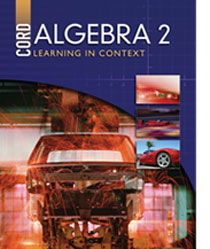Welcome to CORD Online Resources For Learning in Context

Learning in Context is an on-line supplement for our math and science textbooks. Each lesson in CORD’s textbooks is supported by a series of links to various websites. These links add new or more in-depth meaning to the lessons taught in the textbook.

You can navigate each book by using the expanding menu at the left or clicking on the chapters below.

Algebra 2 - First EditionChapters

Chapter 1: Fundamentals of Algebra

Chapter 2: Systems of Equations and Inequalities

Chapter 3: Matrices

Chapter 4: Functions

Chapter 5: Exponents

Chapter 7: Conic Sections

Chapter 8: Exponential and Logarithmic Functions

Chapter 9: Polynomials

Chapter 10: Rational Functions

Chapter 11: Sequences and Series

Chapter 12: Trigonometric Functions

Chapter 13: Trigonometric Graphs and Identities

Chapter 14: Probability